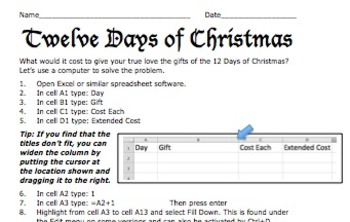# 12 Days of Christmas: A Computer Spreadsheet Activity5th - 9th, Homeschool
Standards
Resource Type
Formats Included
• PDF
Pages
7 pages
\$3.99
List Price:
\$4.99
You Save:
\$1.00
\$3.99
List Price:
\$4.99
You Save:
\$1.00

### Description

This great holiday activity shows students how to use a computer spreadsheet to calculate the cost of their true love's gifts in today's dollars.

The simple step-by-step procedure will allow both beginning and computer-savvy students to experience success as they learn how to use spreadsheet software. Students will enjoy the engaging and entertaining activity.

No computer? No problem! Use the activity master so students can calculate the costs themselves.

Total Pages
7 pages
Included
Teaching Duration
N/A
Report this Resource to TpT
Reported resources will be reviewed by our team. Report this resource to let us know if this resource violates TpT’s content guidelines.

### Standards

to see state-specific standards (only available in the US).
Use variables to represent quantities in a real-world or mathematical problem, and construct simple equations and inequalities to solve problems by reasoning about the quantities.
Identify parts of an expression using mathematical terms (sum, term, product, factor, quotient, coefficient); view one or more parts of an expression as a single entity. For example, describe the expression 2 (8 + 7) as a product of two factors; view (8 + 7) as both a single entity and a sum of two terms.
Write expressions that record operations with numbers and with letters standing for numbers. For example, express the calculation “Subtract 𝘺 from 5” as 5 - 𝘺.
Write, read, and evaluate expressions in which letters stand for numbers.
Write simple expressions that record calculations with numbers, and interpret numerical expressions without evaluating them. For example, express the calculation “add 8 and 7, then multiply by 2” as 2 × (8 + 7). Recognize that 3 × (18932 + 921) is three times as large as 18932 + 921, without having to calculate the indicated sum or product.